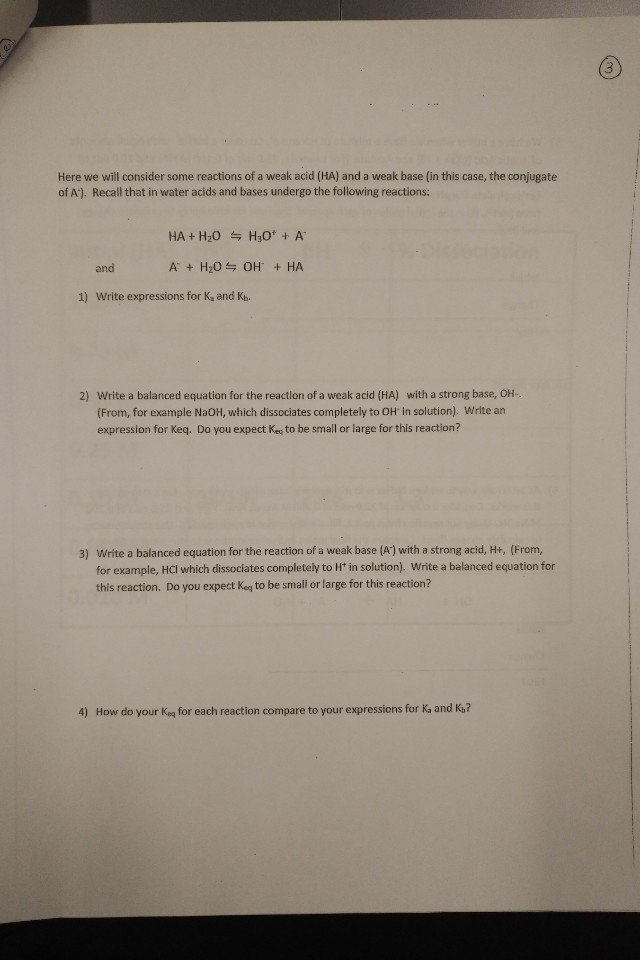# Here we will consider some reactions of a weak acid (HA) and a weak base (in this case, the conjugateof A). Recall that in water acids and bases undergo the following reactions:HA+ H20 H30 + AAH20 OH HAandWrite expressions for K, and Kb1)2) Write a balanced equation for the reaction of a weak acid (HA) with a strong base, OH-(From, for example NaOH, which dissociates completely to OH in solution). Write anexpression for Keq. Do you expect Keg to be small or large for this reaction?3) Write a balanced equation for the reaction of a weak base (A) with a strong acid, H+, (From,for example, HCl which dissociates completely to Ht in solution). Write a balanced equation forthis reaction. Do you expect Keg to be small or large for this reaction?4)How do your Keg for each reaction compare to your expressions for Ka and Kb?

Question
94 views

On problem 2, how can i expect that Keq to be small or large for this reaction?help_outlineImage TranscriptioncloseHere we will consider some reactions of a weak acid (HA) and a weak base (in this case, the conjugate of A). Recall that in water acids and bases undergo the following reactions: HA+ H20 H30 + A AH20 OH HA and Write expressions for K, and Kb 1) 2) Write a balanced equation for the reaction of a weak acid (HA) with a strong base, OH- (From, for example NaOH, which dissociates completely to OH in solution). Write an expression for Keq. Do you expect Keg to be small or large for this reaction? 3) Write a balanced equation for the reaction of a weak base (A) with a strong acid, H+, (From, for example, HCl which dissociates completely to Ht in solution). Write a balanced equation for this reaction. Do you expect Keg to be small or large for this reaction? 4) How do your Keg for each reaction compare to your expressions for Ka and Kb? fullscreen
check_circle

Step 1

The equilibrium constant for this reaction will be used to determine the extent of the given reaction. The acid dissociation which is denoted by the symbol Ka can be determined from the product of the equilibrium constant for this reaction and the concentration of the water.

Step 2

The balanced chemical equation which shows the chemical reaction that takes place between a weak acid HA and a strong base say NaOH, which completely dissociates to its constituent ions is shown below:

Step 3

The expression for the equilibrium constant for ...

### Want to see the full answer?

See Solution

#### Want to see this answer and more?

Solutions are written by subject experts who are available 24/7. Questions are typically answered within 1 hour.*

See Solution
*Response times may vary by subject and question.
Tagged in

### Physical Chemistry